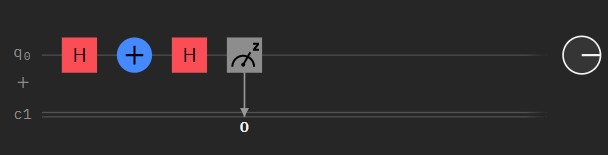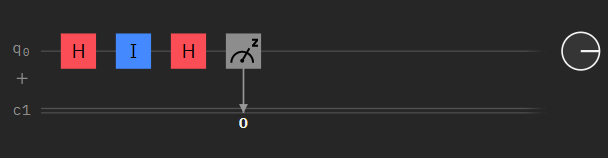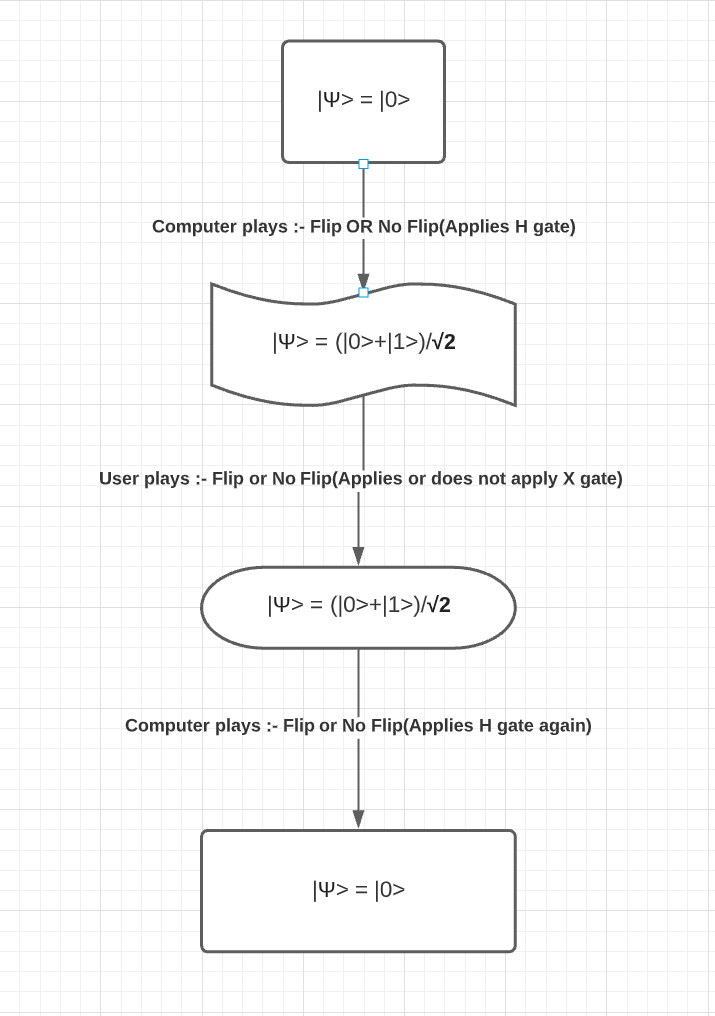# Quantum coins

Quantum coins is a game intended to show some of the fundamental properties of a quantum computer, which are superposition and uncertinity. The most basic unit of information in a classical computer is a bit (0 or 1).A quantum computer on the other hand uses qubit which has a more fluid nature than a bit. A qubit can simultaneously be 0 and 1 with some probability of being zero and some probability of being 1. A superposition is a property of a qubit to be in a fluid nature, which states that the state of the coin can be something other that 0 or 1, which in our case lies somewhere in between 0 and 1. The measurement of the quantum state in superposition leads to the collapse of the quantum state to either 0 or 1. This phenomenon is called Wave Function Collapse. The result of the collapse is stored in a classical bit C0, C1 etc. A superposition is created in a qubit by using a Hadamard Gate. A classical computer cannot create a bit that has a fluid nature. A bit is limited to its 0 and 1 state. To carry out operations in a classical computer various gates are used like the AND gate, OR gate, NOT gate, XOR gate etc. The NOT gate, also called a bit flip gate, converts the 0 stae to 1 state and 1 state to 0 state. A bit flip however has no effect on a superposition of a quantum state. This is the working principal of our game. Moreover when two H gates act on a single qubit we get a matrix which when applied to a state 0 or 1 does not change the input, as it forms an identity matrix. When a superposition is created and a bit flip is applied to it, superposition is not affected, when another H gate is applied the initial state of the qubit is recovered.

# Our Proposal

We do not get into the process of the computer making a move, we straight away apply the H gate, for both the moves of the computer. The user will have the choice to apply the bit flip operator. The result will be that no matter what the user does, the quantum state of coin is not affected.

The Hadamard matrix is given as follows :

$\begin{pmatrix} 1/\sqrt2 & 1/\sqrt2\\ 1/\sqrt2 & -1/\sqrt2 \end{pmatrix}$

Our initial quantum sate is |0> which i9s represented by : $$\begin{pmatrix} 1\\ 0 \end{pmatrix}$$

The bit flip gate is given by :

$\begin{pmatrix} 0 & 1\\ 1 & 0 \end{pmatrix}$

$$H | 0>$$ we get the $$| +>$$ state. When we apply the X gate to the | +> state, the | +> state is not affected. When another H gate is applied to the qubit, the state collapses to | 0>. When we measure the state we get a 100% the | 0> state.

### Pre-requisites

For its proof of concept, the Qiskit framework will be used for the construction of the quantum circuit.

So, the initial state of coin (or by its abbreviation isoc) is kept Heads.

• First the Computer applies the H gate on the circuit.
• Next the players input is taken and the circuit is modified accordingly.
• At the end the computer applies the H gate again on the circuit and then the measurement is made.
• The game requires atleast a 1 Qubit processor(ex.:- ibmq_armonk) or higher.
# Importing standard Qiskit libraries and configuring account
import qiskit
from qiskit import *
from qiskit.tools.visualization import plot_histogram
import matplotlib.pyplot as plt
from qiskit import IBMQ
# importing IBM account
IBMQ_account_id = '' # Your IBMQ API Token is to be assigned to the IBMQ_account_id variable.
'''
An IBMQ API Token is a 128 bit long alphanumeric string which is used to access your IBMQ systems
on cloud. Absence of an API Token will not load and save your IBMQ account and hence the provider
ibm-q used in section 2 will raise an error. Using the API Token once and regenerating it will stop
all ascess to applications with your current API Token.
'''
IBMQ.save_account(IBMQ_account_id)

circuit = QuantumCircuit(1,1)
'''
Creating a Quantum circuit with 1 Qubit and 1 classical bit, the classical bit
is used to store the measurement result of the Qubit.
'''
circuit.h(0) # Adding H Gate to the circuit (Computers initial move)
i = input("Choose to 'Flip' or 'Do not flip' the coin :-  ") #ui1
'''
The following if-else block applies the necessary gates on the circuit.
For "Flip" decision by the user an X gate is applied, and for "Not Flip"
decision by the user an identity is applied.
'''
if i == 'Flip':
circuit.x(0)
elif i == 'Do not flip':
circuit.i(0)
else:
# For any other decision a exception "Incorrect Choice" is raised by the computer.
raise Exception('Incorrect choice')
circuit.h(0) # Computers final move
circuit.measure(0,0) # Final measurement on circuit is applied.
'''
ibm-q is used a default as the default provider. Again it will not work if your
IBMQ account is not saved and loaded. We are using ibmqx2 is used as the default
however ibmq_armonk(a canary r1.2 processor) is the preffered processor and backend.
IBMQ_armonk is a 1 Qubit processor with 1 Quantum volume.
'''
provider = IBMQ.get_provider(hub='ibm-q')
backend = provider.get_backend('ibmqx2')
exe = execute(circuit, backend, shots=1).result() # Circuit is executed with only 1 measurement.
counts = exe.get_counts() # Counts are calculated.
'''
Again, sometimes the result is not the |0> state but the |1> state, which makes the player win.
When we run the circuit is run on a real quantum computer due to noise in the enviornment
and operational errors the result is |1>. Hence the player wins. As we improve our hardware the
result will move towards the ideal result.
'''
for i in counts: # The counts dictionary is itrated and the result is printed.
if i == '0':
print('The computer won.')
else:
print('You win.')
# plot_histogram(s), a histogram can be plotted of the outcomes to show computer vs player win count.

Choose to 'Flip' or 'Do not flip' the coin :-  Flip
The computer won.


# Logic Circuit digram for each case

##### for flip choice by the user##### for not to flip choice by the userIf played on a classical computer the player has a 50% chance of winning against the computer. When the same idea is imposed on a Quantum Circuit the results are completely in favour of the computer, the computer wins 100% of times. The game uses the 2 qubit version of the Out0 algorithm. Through out the course of the game there are three moves, two by the computer and one by the user. The process however involves nearly 6 choices to be, made either to flip or not. The game begins with a coin initially in Heads state. The Heads state is represented by the |0> quantum state. The Quantum Computer exhibits the property of superposition by using the H gate. The computer applies a H gate which creates the |+> state, user as always has the choice to flip the bit or not by applying the X gate. There are certain particularities that are achieved in qubits by means of quantum gates. One interesting relationship that we apply is with HXH gates. Although a superposition is initalized, the three gates used to achieve the superposition are equivalent to the Z gate. The result is that the state it does not change, it will always give us |0>, so the quantum computer will always win. To demonstration this, we will apply the quantum circuit and will use as backend as ibmqx2 to apply the quantum circuit for the game. Hence not matter what the user does, flip or no flip, the computer always wins.

## Brief answer’s to important questions

1 –> What happens when you apply the Hadamard gate on the coin?

A Hadamard gate is gate which creates an equal superposition of the $$| 0>$$ and $$| 1>$$ state which we call the $$| +>$$ state. We say that the H gate creates a quantum state which is a linear combination of the states $$| 0>\text{ and } | 1>$$.

$|\psi> = \alpha|0> + \beta|1>$

Here $$\alpha^2$$ and $$\beta^2$$ is the probability of measuring $$| 0>\text{ and } | 1>$$ respectively.

Here $$\alpha^2 = \beta^2 = 0.5 \text{ and } \alpha = \beta = 1/\sqrt2$$

2 –> What happens if you apply X to the coin?

The X gate is the bit flip gate. For example :-

X(0) -> 1

X(1) -> 0

When a bit flip is applied to a Heads state, it changes it to Tales and if bit flip is applied on Tales it gives the Heads state.

3 –> What happens if you apply Hadamard and X to the coin?

When a Hadamard gate is applied to a $$| 0>$$ quantum state it changes to $$| +>$$ the X gate however has no effect on the superposition and hence the $$| +>$$ state remains unaffected.

4 –> What happens if you apply Hadamard, X, and Hadamard to the coin?

As we said, when a superposition is created, the X gate has no affect on the superposition. When another H gate is applied the resultant is an Identity which gives $$| 0>$$ as the initial state was zero.

5 –> What happens if you apply X and Hadamard to the coin?

When the $$X$$ gate is applied and then H gate is applied, the result is different that usual. The initial state is :

$\begin{pmatrix} 1\\ 0 \end{pmatrix}$

The $$X$$ gate on the initial state gives the state $$| 1>$$ :

$\begin{pmatrix} 0\\ 1 \end{pmatrix}$

When hadamard gate on $$| 1>$$ gives what we call the $$| ->$$ state, given by:

$|\psi> = (|0> - |1>)/\sqrt2$

There is still $$0.5$$ probability of $$|0>$$ and $$0.5$$ probability of $$-|b1>$$.

6 –> What happens when the circuit is executed n times?

As per the logic circuit diagram any input will give a 100% output of $$| 0>$$ . The complexity the algorithm in Big-O notation is O(1) because executing circuit for flip takes the same time as circuit for not flip.

## Here is a chart that explains the process of the game.(As per second proposal)